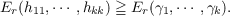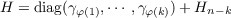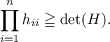#### Vol. 34, No. 2, 1970

 Download this articleFor screen For printingRecent Issues Vol. 325: 1  2 Vol. 324: 1  2 Vol. 323: 1  2 Vol. 322: 1  2 Vol. 321: 1  2 Vol. 320: 1  2 Vol. 319: 1  2 Vol. 318: 1  2Online Archive Volume: Issue:The Journal Subscriptions Editorial Board Officers Contacts Submission Guidelines Submission Form Policies for Authors ISSN: 1945-5844 (e-only) ISSN: 0030-8730 (print) Special Issues Author Index To Appear Other MSP Journals
An analysis of equality in certain matrix inequalities. I

### William R. Gordon and Marvin David Marcus

Vol. 34 (1970), No. 2, 407–413
##### Abstract

In this paper we are concerned with analyzing the cases of equality in certain inequalities that relate the eigenvalues and main diagonal elements of hermitian matrices.

Let Er denote the r-th elementary symmetric function of k variables (E0 = 1). If H = (hτj) is an n-square positive semidefinite hermitian matrix with eigenvalues γ1 γn and if 1 r k n, then it is known that(1.1)

If r > 1 and at least r of h11,,hkk are positive then (1.1) can be equality if and only if there exists a permutation φ lSk such that(1.2)

where Hnk is (nk)-square and + denotes direct sum. Of course, if r = k = n then (1.1) is the Hadamard determinant theorem:(1.3)

If some hii = 0, then H is singular and (1.3) is equality. If hii > 0,i = 1,,n, then the condition (1.2) yields the well-known criterion for equality in (1.3), namely H = diag(h11,,hnn).

Primary: 15.55
##### Milestones